/

### The Kumaraswamy distribution

The Kumaraswamy distribution describes a distribution in which outcomes are limited to a specific range, the probability density function within this range being characterised by two shape parameters. It is similar to the beta distribution but possibly easier to use because it has simpler analytical expressions for its probability density function and cumulative distribution function.

 Distribution name Kumaraswamy distribution Common notation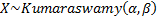Parameters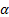= shape parameter (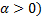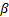= shape parameter (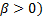Domain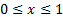Probability density function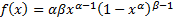Cumulative distribution function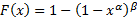Mean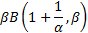Variance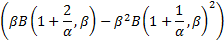Other comments A standard Kumaraswamy distribution has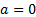and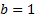. Its non-central moments are given by: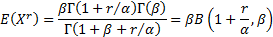and its median is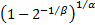and its mode is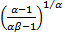(for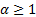,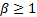,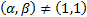.

Nematrian web functions

Functions relating to the above distribution may be accessed via the Nematrian web function library by using a DistributionName of “kumaraswamy”. Functions relating to a generalised version of this distribution including additional location (i.e. shift) and scale parameters may be accessed by using a DistributionName of “kumaraswamy4” ”, see also including additional shift and scale parameters. For details of other supported probability distributions see here.